Search
Document Center

# Time series

Last Updated: Jun 01, 2020

## x13_arima

• Autoregressive Integrated Moving Average Model (ARIMA) is a famous time series prediction method defined by Box and Jenkins in the early 1970s. Therefore, this model is also called the Box-Jenkins model or the Box-Jenkins approach.

• x13-arima is an ARIMA algorithm based on the open-source X-13ARIMA-SEATS seasonal adjustment.

#### PAI command

``PAI -name x13_arima``    -project algo_public``    -DinputTableName=pai_ft_x13_arima_input``    -DseqColName=id ``    -DvalueColName=number``    -Dorder=3,1,1``    -Dstart=1949.1``    -Dfrequency=12``    -Dseasonal=0,1,1``    -Dperiod=12``    -DpredictStep=12``    -DoutputPredictTableName=pai_ft_x13_arima_out_predict``    -DoutputDetailTableName=pai_ft_x13_arima_out_detail``

#### Parameter description

Parameter Description Value range Required/Optional, default value/act
inputTableName Input table Table name Required
inputTablePartitions Partitions used for training in the input table, in the format of partition_name=value. The multilevel format is name1=value1/name2=value2. Multiple partitions are separated by commas (,). Partition name (Optional) All partitions are selected by default.
seqColName Time series column Column name (Required) It is used only to sort valueColNames, and the value is irrelevant to the algorithm.
valueColName Value column Column name Required
groupColNames Grouping column. Multiple columns are separated by commas (,), such as col0,col1. A time series is created for each group. Column name Optional
order p, d, and q respectively represent the autoregressive coefficient, difference, and moving regression coefficient. p, d, and q are non-negative integers in the range of [0, 36]. Required
start Time series start date String, in the format of year.seasonal, such as 1986.1 Optional; default value: 1.1
frequency Frequency of time series Positive integer in the range of (0, 12] Optional; default value: 12, indicating 12 months/year
seasonal sp, sd, and sq respectively represent the seasonal autoregressive coefficient, seasonal difference, and seasonal moving regression coefficient. sp, sd, and sq are all non-negative integers in the range of [0, 36]. Optional; default value: not seasonal
period Seasonal period Number in the range of (0, 100] Optional; default value: frequency
maxiter Maximum number of iterations Positive integer Optional; default value: 1500
tol Tolerance Double type Optional; default value: 1e-5
predictStep Number of prediction items Number in the range of (0, 365] Optional; default value: 12
confidenceLevel Prediction confidence level Number in the range of (0, 1) Optional; default: 0.95
outputPredictTableName Prediction output table name Table name Required
outputDetailTableName Detail table Table name Required
outputTablePartition Partitions in the output table Partition name Optional, not output to the partition by default
coreNum Number of nodes Paired with the memSizePerCore parameter, positive integer (Optional) Automatically calculated by default
memSizePerCore Memory size of each node, in MB Positive integer in the range of [1024, 64*1024] (Optional) Automatically calculated by default
lifecycle (Optional) Lifecycle of the output table Positive integer No lifecycle

#### Time series format

The start and frequency parameters specify the two time dimensions of data (valueColName), TS1 and TS2. The frequency parameter represents the data frequency within a cycle, namely, the frequency of TS2 in each TS1. The start parameter is in the format of n1.n2, which indicates that the start date is the N2 TS2 in the N1 TS1.

Unit time ts1 ts2 frequency start
12 months/Year Year Month 12 1949.2 represents the 2nd month of the 1949th year.
Four seasons/year Year Season 4 1949.2 represents the second quarter of the 1949th year.
Seven days/week Week Day 7 1949.2 represents the second day of the 1949th week.
1 Any time unit 1 1 1949.1 represents the 1949th (year, day, hour, and so on).

For example, value=[1,2,3,5,6,7,8,9,10,11,12,13,14,15]

• start=1949.3, frequency=12 indicates that the data frequency is monthly per year, and the prediction start date is 1950.06.
year Jan Feb Mar Apr May Jun Jul Aug Sep Oct Nov Dec
1949 1 2 3 4 5 6 7 8 9 10
1950 11 12 13 14 15
• start=1949.3, frequency=4 indicates that the data frequency is quarterly per year, and the prediction start date is 1953.02.
year Qtr1 Qtr2 Qtr3 Qtr4
1949 1 2
1950 3 4 5 6
1951 7 8 9 10
1952 11 12 13 14
1953 14
• start=1949.3, frequency=7 indicates that the data frequency is daily per week, and the prediction start date is 1951.04.
week Sun Mon Tue Wed Thu Fri Sat
1949 1 2 3 4 5
1950 6 7 8 9 10 11 12
1951 13 14 15
• start=1949.1, frequency=1 can represent any time unit, and the prediction start date is 1963.00.
cycle p1
1949 1
1950 2
1951 3
1951 4
1952 5
1953 6
1954 7
1955 8
1956 9
1957 10
1958 11
1959 12
1960 13
1961 14
1962 15

#### Example

Test data

Used data: AirPassengers.

This data set is the number of passengers for international airlines each month from 1949 to 1960, as shown in the following table.

id number
1 112
2 118
3 132
4 129
5 121

Upload data by tuunel. The command is as follows.

``create table pai_ft_x13_arima_input(id bigint,number bigint);``tunnel upload data/airpassengers.csv pai_ft_x13_arima_input -h true;``

PAI command

``PAI -name x13_arima``    -project algo_public``    -DinputTableName=pai_ft_x13_arima_input``    -DseqColName=id ``    -DvalueColName=number``    -Dorder=3,1,1``    -Dseasonal=0,1,1``    -Dstart=1949.1``    -Dfrequency=12``    -Dperiod=12``    -DpredictStep=12``    -DoutputPredictTableName=pai_ft_x13_arima_out_predict``    -DoutputDetailTableName=pai_ft_x13_arima_out_detail``

Output description

• Output table: outputPredictTableName.
The fields are:
column namecomment
pdatePrediction date
forecastPrediction conclusion
lowerLower threshold of the prediction conclusion when the confidence level is confidenceLevel (default: 0.95)
upperUpper threshold of the prediction conclusion when the confidence level is confidenceLevel (default: 0.95)
Data display: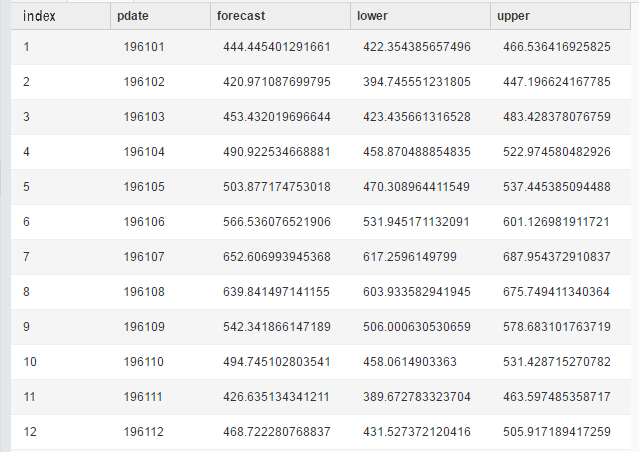• Output table: outputDetailTableName.
The fields are:

column namecomment
keymodel: indicates the model.
evaluation: indicates the evaluation result.
parameters: indicates training parameters.
log: indicates the training log.
summaryStorage details
Data display: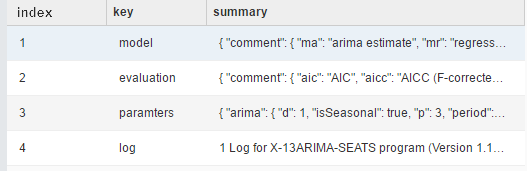Display on the PAI web - Model factor (key=model)Display on the PAI web - Evaluation index (key=evaluation)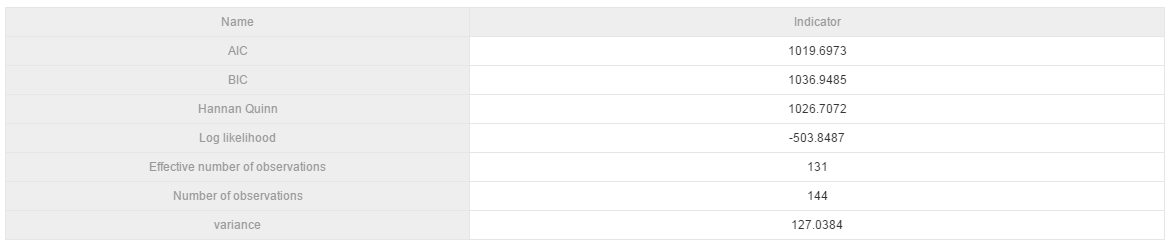#### Algorithm scale

• Supported scale

• Rows: a maximum of 1200 rows in a single group

• Columns: 1 data column

• Resource calculation method

• Default calculation method used when groupColNames is not set
coreNum = 1
memSizePerCore = 4096

• Default calculation method used when groupColNames is set
coreNum = floor (total number of data rows / 120,000)
memSizePerCore = 4096

## x13_auto_arima

x13-auto-arima includes an automatic ARIMA model selection procedure based largely on the procedure of Gomez and Maravall (1998) as implemented in TRAMO (1996) and subsequent revisions.

The x13-auto-arima selection process is as follows:

1. Default model estimation.

• When frequency is 1, the default model is (0,1,1).
• When frequency is greater than 1, the default model is (0,1,1)(0,1,1).
2. Identication of dierencing orders.

• Skip this step if you set diff and seasonalDiff.
• Determine the difference d and the seasonal difference D by using the Unit root test (wiki ).
3. Identication of ARMA model orders.

Select the optimal model based on BIC(wiki), and the maxOrder and maxSeasonalOrder parameters are used in this step.

4. Comparison of identified model with default model.

Compare models by using Ljung-Box Q statistic(wiki). If both models are unacceptable, use the (3,d,1)(0,D,1) model.

5. Final model checks.

#### PAI command line

``PAI -name x13_auto_arima``    -project algo_public``    -DinputTableName=pai_ft_x13_arima_input``    -DseqColName=id ``    -DvalueColName=number``    -Dstart=1949.1``    -Dfrequency=12``    -DpredictStep=12``    -DoutputPredictTableName=pai_ft_x13_arima_out_predict2``    -DoutputDetailTableName=pai_ft_x13_arima_out_detail2``

#### Parameter description

Parameter Description Value range Required/Optional, default value/act
inputTableName Input table Table name Required
inputTablePartitions Partitions used for training in the input table, in the format of partition_name=value. The multilevel format is name1=value1/name2=value2. Multiple partitions are separated by commas (,). Partition name (Optional) All partitions are selected by default.
seqColName Time series column Column name (Required) It is used only to sort valueColNames, and the value is irrelevant to the algorithm.
valueColName Value column Column name Required
groupColNames Grouping column. Multiple columns are separated by commas (,), such as col0,col1. A time series is created for each group. Column name Optional
start Time series start date String, in the format of year.seasonal, such as 1986.1 Optional; default value: 1.1
frequency Frequency of time series Positive integer in the range of (0, 12] Optional; default value: 12, indicating 12 months/year
maxOrder Maximum values of p and q Non-negative integer in the range of [0, 4] Optional; default value: 2
maxSeasonalOrder Maximum values of seasonal p and q Non-negative integer in the range of [0, 2] Optional; default value: 1
maxDiff Maximum value of differential d Non-negative integer in the range of [0, 2] Optional; default value: 2
maxSeasonalDiff Maximum value of seasonal differential d Non-negative integer in the range of [0,1] Optional; default value: 1
diff Differential d Non-negative integer in the range of [0, 2]
When both diff and maxDiff are set, maxDiff is ignored.
diff and seasonalDiff must be both set.
Optional; default value: -1; no diff specified
seasonalDiff Seasonal differential d Non-negative integer in the range of [0, 1]
When both seasonalDiff and maxSeasonalDiff are set,
maxSeasonalDiff is ignored.
Optional; default value: -1; no seasonalDiff specified
maxiter Maximum number of iterations Positive integer Optional; default value: 1500
tol Tolerance Double type Optional; default value: 1e-5
predictStep Number of prediction items Number in the range of (0, 365] Optional; default value: 12
confidenceLevel Prediction confidence level Number in the range of (0, 1) Optional; default: 0.95
outputPredictTableName Prediction output table name Table name Required
outputDetailTableName Detail table Table name Required
outputTablePartition Partitions in the output table Partition name Optional, not output to the partition by default
coreNum Number of nodes Paired with the memSizePerCore parameter, positive integer (Optional) Automatically calculated by default
memSizePerCore Memory size of each node, in MB Positive integer in the range of [1024, 64*1024] (Optional) Automatically calculated by default
lifecycle (Optional) Lifecycle of the output table Positive integer No lifecycle

#### Time series format

The start and frequency parameters specify the two time dimensions of data (valueColName), TS1 and TS2.The frequency parameter represents the data frequency within a cycle, namely, the frequency of TS2 in each TS1. The start parameter is in the format of n1.n2, which indicates that the start date is the N2 TS2 in the N1 TS1.

Unit time ts1 ts2 frequency start
12 months/Year Year Month 12 1949.2 represents the 2nd month of the 1949th year.
Four seasons/year Year Season 4 1949.2 represents the second quarter of the 1949th year.
Seven days/week Day Week 7 1949.2 represents the second day of the 1949th week.
1 Any time unit 1 1 1949.1 represents the 1949th (year, day, hour, and so on).

For example, value=[1,2,3,5,6,7,8,9,10,11,12,13,14,15]

• start=1949.3, frequency=12 indicates that the data frequency is monthly per year, and the prediction start date is 1950.06.
year Jan Feb Mar Apr May Jun Jul Aug Sep Oct Nov Dec
1949 1 2 3 4 5 6 7 8 9 10
1950 11 12 13 14 15
• start=1949.3, frequency=4 indicates that the data frequency is quarterly per year, and the prediction start date is 1953.02.
year Qtr1 Qtr2 Qtr3 Qtr4
1949 1 2
1950 3 4 5 6
1951 7 8 9 10
1952 11 12 13 14
1953 14
• start=1949.3, frequency=7 indicates that the data frequency is daily per week, and the prediction start date is 1951.04.
week Sun Mon Tue Wed Thu Fri Sat
1949 1 2 3 4 5
1950 6 7 8 9 10 11 12
1951 13 14 15
• start=1949.1, frequency=1 can represent any time unit, and the prediction start date is 1963.00.
cycle p1
1949 1
1950 2
1951 3
1951 4
1952 5
1953 6
1954 7
1955 8
1956 9
1957 10
1958 11
1959 12
1960 13
1961 14
1962 15

#### Example

Test data

Used data: AirPassengers

This data set is the number of passengers for international airlines each month from 1949 to 1960, as shown in the following table.

id number
1 112
2 118
3 132
4 129
5 121

Upload data by tuunel. The command is as follows.

``create table pai_ft_x13_arima_input(id bigint,number bigint);``tunnel upload data/airpassengers.csv pai_ft_x13_arima_input -h true;``

PAI command

``PAI -name x13_auto_arima``    -project algo_public``    -DinputTableName=pai_ft_x13_arima_input``    -DseqColName=id ``    -DvalueColName=number``    -Dstart=1949.1``    -Dfrequency=12``    -DmaxOrder=4``    -DmaxSeasonalOrder=2``    -DmaxDiff=2``    -DmaxSeasonalDiff=1``    -DpredictStep=12``    -DoutputPredictTableName=pai_ft_x13_arima_auto_out_predict``    -DoutputDetailTableName=pai_ft_x13_arima_auto_out_detail``

Output description

• Output table: outputPredictTableName.
The fields are:
column namecomment
pdatePrediction date
forecastPrediction conclusion
lowerLower threshold of the prediction conclusion when the confidence level is confidenceLevel (default: 0.95)
upperUpper threshold of the prediction conclusion when the confidence level is confidenceLevel (default: 0.95)
Data display: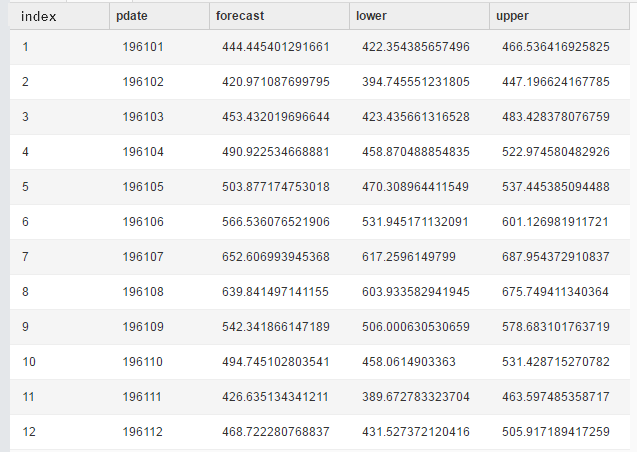• Output table: outputDetailTableName.

The fields are:

column namecomment
keymodel: indicates the model.
evaluation: indicates the evaluation result.
parameters: indicates training parameters.
log: indicates the training log.
summaryStorage details
Data display: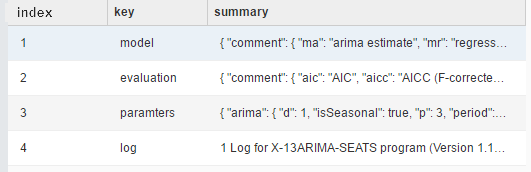Display on the PAI web - Model factor (key=model)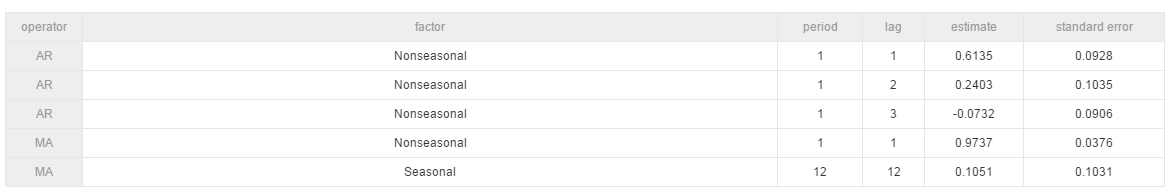Display on the PAI web - Evaluation index (key=evaluation)#### Algorithm scale

• Supported scale

• Rows: a maximum of 1200 rows in a single group

• Columns: 1 data column

• Resource calculation method

• Default calculation method used when groupColNames is not set
coreNum = 1
memSizePerCore = 4096

• Default calculation method used when groupColNames is set
coreNum = floor (total number of data rows / 120,000)
memSizePerCore = 4096

#### Important notes

Why are the prediction results the same?

When an exception occurs during model training, the mean model is called, and all prediction results are the mean of the training data.

Common exceptions include “Not stationary after timing differential diff”, “training does not converge”, “variance is 0”. You can view the stderr file of individual nodes in the logview to obtain exception details.